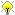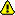[Documentation] [TitleIndex] [WordIndex]

 Note: This tutorial assumes that you have completed the previous tutorials: Communicating with a realtime joint controller.Please ask about problems and questions regarding this tutorial on answers.ros.org. Don't forget to include in your question the link to this page, the versions of your OS & ROS, and also add appropriate tags.

# Adding a PID to a realtime joint controller

Description: This tutorial teaches you how to add a PID object to a realtime joint controller

Tutorial Level: INTERMEDIATE

Next Tutorial: Capturing data from a controller

## Introduction

This tutorial builds on the controller we created in the previous tutorial. Here we will start using a PID object inside the controller. The PID controller is part of the control_toolbox package.

### The code

Now we need to add the pid controller to our controller code. The resulting code is shown below:

include/my_controller_pkg/my_controller_file.h

```   1 #include <pr2_controller_interface/controller.h>
2 #include <pr2_mechanism_model/joint.h>
3 #include <ros/ros.h>
4 #include <my_controller_pkg/SetAmplitude.h>
5 #include <control_toolbox/pid.h>
6
7
8 namespace my_controller_ns{
9
10 class MyControllerClass: public pr2_controller_interface::Controller
11 {
12 private:
13   bool setAmplitude(my_controller_pkg::SetAmplitude::Request& req,
14                     my_controller_pkg::SetAmplitude::Response& resp);
15
16   pr2_mechanism_model::JointState* joint_state_;
17   double init_pos_;
18   double amplitude_;
19   ros::ServiceServer srv_;
20   control_toolbox::Pid pid_controller_;
21   pr2_mechanism_model::RobotState *robot_;
22   ros::Time time_of_last_cycle_;
23
24 public:
25   virtual bool init(pr2_mechanism_model::RobotState *robot,
26                     ros::NodeHandle &n);
27   virtual void starting();
28   virtual void update();
29   virtual void stopping();
30 };
31 }
```

Compared to the original code, the header changed in the following places:

```   5 #include <control_toolbox/pid.h>
6
```

Here we include the pid controller and a gains setter that will allow us to change to controller gains on the fly using service calls. Very similar to the service we added to change the amplitude of the sinusoid on the fly.

```  20   control_toolbox::Pid pid_controller_;
21   pr2_mechanism_model::RobotState *robot_;
22   ros::Time time_of_last_cycle_;
```

Here we add a class variable to store

• the pid object,
• a pointer to the robot model (this is the pointer that is given to us in the init(..) method, and
• the last time the controller update loop ran. With this time we can compute the delta time between two consecutive runs of the update loop.

Next, here is the new code for the cpp file: src/my_controller_file.cpp

```   1 #include "my_controller_pkg/my_controller_file.h"
2 #include <pluginlib/class_list_macros.h>
3
4 using namespace my_controller_ns;
5
6
7 /// Controller initialization in non-realtime
8 bool MyControllerClass::init(pr2_mechanism_model::RobotState *robot,
9                              ros::NodeHandle &n)
10 {
11   // get joint name
12   std::string joint_name;
13   if (!n.getParam("joint_name", joint_name))
14   {
15     ROS_ERROR("No joint given in namespace: '%s')",
16               n.getNamespace().c_str());
17     return false;
18   }
19
20   // get pointer to joint state
21   joint_state_ = robot->getJointState(joint_name);
22   if (!joint_state_)
23   {
24     ROS_ERROR("MyController could not find joint named '%s'",
25               joint_name.c_str());
26     return false;
27   }
28
29   // advertise service to set amplitude
30   amplitude_ = 0.5;
32                             &MyControllerClass::setAmplitude, this);
33
34
35   // copy robot pointer so we can access time
36   robot_ = robot;
37
38   // construct pid controller
39   if (!pid_controller_.init(ros::NodeHandle(n, "pid_parameters"))){
40     ROS_ERROR("MyController could not construct PID controller for joint '%s'",
41               joint_name.c_str());
42     return false;
43   }
44
45   return true;
46 }
47
48
49 /// Controller startup in realtime
50 void MyControllerClass::starting()
51 {
52   init_pos_ = joint_state_->position_;
53   time_of_last_cycle_ = robot_->getTime();
54   pid_controller_.reset();
55 }
56
57
58 /// Controller update loop in realtime
59 void MyControllerClass::update()
60 {
61   double desired_pos = init_pos_ + amplitude_ * sin(ros::Time::now().toSec());
62   double current_pos = joint_state_->position_;
63
64   ros::Duration dt = robot_->getTime() - time_of_last_cycle_;
65   time_of_last_cycle_ = robot_->getTime();
66   joint_state_->commanded_effort_ = pid_controller_.updatePid(current_pos-desired_pos, dt);
67 }
68
69
70 /// Controller stopping in realtime
71 void MyControllerClass::stopping()
72 {}
73
74
75 /// Service call to set amplitude of sin
76 bool MyControllerClass::setAmplitude(my_controller_pkg::SetAmplitude::Request& req,
77                                      my_controller_pkg::SetAmplitude::Response& resp)
78 {
79   if (fabs(req.amplitude) < 2.0){
80     amplitude_ = req.amplitude;
81     ROS_INFO("Mycontroller: set amplitude too %f", req.amplitude);
82   }
83   else
84     ROS_WARN("MyController: requested amplitude %f too large", req.amplitude);
85
86   resp.amplitude = amplitude_;
87   return true;
88 }
89
90
91 /// Register controller to pluginlib
92 PLUGINLIB_REGISTER_CLASS(MyControllerPlugin,
93                          my_controller_ns::MyControllerClass,
94                          pr2_controller_interface::Controller)
```

The changes in the cpp file are:

```  35   // copy robot pointer so we can access time
36   robot_ = robot;
```

Here we store a copy to the robot. The robot object is used to get the time at which the controllers are triggered. This time is different from ros::Time::now()! The time provided by the robot model is the same for all controllers, and is the time at which the controller manager started triggering all controllers.

```  38   // construct pid controller
39   if (!pid_controller_.init(ros::NodeHandle(n, "pid_parameters"))){
40     ROS_ERROR("MyController could not construct PID controller for joint '%s'",
41               joint_name.c_str());
42     return false;
43   }
```

Here we initialize the new pid controller. The init method takes a NodeHandle that specifies the namespace in which the pid parameters are specified. In this case, the namespace is the controller name plus the string "pid_parameters". This namespace is important to set the pid values in our launch file.

```  53   time_of_last_cycle_ = robot_->getTime();
54   pid_controller_.reset();
```

In the starting method of the controller we store the controller time, so we can later compute the difference in time between two update loops.

```  60 {
61   double desired_pos = init_pos_ + amplitude_ * sin(ros::Time::now().toSec());
62   double current_pos = joint_state_->position_;
63
64   ros::Duration dt = robot_->getTime() - time_of_last_cycle_;
65   time_of_last_cycle_ = robot_->getTime();
66   joint_state_->commanded_effort_ = pid_controller_.updatePid(current_pos-desired_pos, dt);
67 }
```

This is the place we actually use the pid controller. The pid needs an error signal, plus the time interval. First we compute the time interval "dt", and then we compute the error signal as the difference between the measured and desired position.Note that the pid controller uses negative feedback (error = actual - desired)

### Configuration

To compile this new code, you should add the control_toolbox package as a dependency to your package. Add the following line to your manifest.xml:

` <depend package="control_toolbox"/>`

So now we're ready to build the controller. Because we just added a dependency, and we want to be sure this dependency gets build too, we use "rosmake" instead of just "make"

`  \$ rosmake`

Next, we should add the parameters for the pid to the yaml file my_controller.yaml:

```  my_controller_name:
type: MyControllerPlugin
joint_name: r_shoulder_pan_joint
pid_parameters:
p: 10.0
i: 0.0
d: 0.0
i_clamp: 0.0```

## Running the controller

As before, the new code has to get loaded into the realtime process. So you can either restart all of gazebo with:

```  \$ roslaunch gazebo_worlds empty_world.launch
\$ roslaunch pr2_gazebo pr2.launch```

OR if gazebo is still running, you can use the pr2_controller_manager to request a reload of the libraries:

`  \$ rosrun pr2_controller_manager pr2_controller_manager reload-libraries`

And now, launch our new controller:

`  \$ roslaunch my_controller.launch`

2020-01-25 12:59